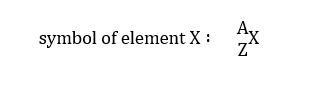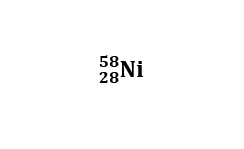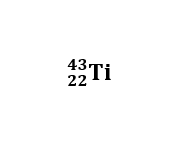# Write the complete symbol, including mass number and atomic number, for each atom.contains 28 protons28 protons and 30 neutrons30 neutrons:5828NiNi2858contains 22 protons22 protons and 21 neutrons21 neutrons:4322TiTi2243contains 15 electrons15 electrons and 19 neutrons19 neutrons an oxygen atom with 10 neutrons10 neutrons:188OO818a chromium atom with a mass number of 5454:

Question
220 views
Write the complete symbol, including mass number and atomic number, for each atom.
contains 28 protons28 protons and 30 neutrons30 neutrons:
5828NiNi2858
contains 22 protons22 protons and 21 neutrons21 neutrons:
4322TiTi2243
contains 15 electrons15 electrons and 19 neutrons19 neutrons

an oxygen atom with 10 neutrons10 neutrons:
188OO818
a chromium atom with a mass number of 5454:
check_circle

Introduction:

Atomic number: The atomic number (represented by the letter Z) of an element is the number of protons in the nucleus of each atom of that element.

Mass number: The mass number (represented by the letter A) is defined as the total number of protons and neutrons in an atom.

The composition of any atom can be illustrated with a shorthand notation called A/Z format. Both the atomic number and mass are written to the left of the chemical symbol. The "A" value is written as a superscript while the "Z" value is written as a subscript.

Mass number = number of protons + number of neutrons.

Symbol of elements.1. Writing the symbol for an element with 28 protons and 30 neutrons:

Atomic number (Z) = 28

Mass number = number of protons + number of neutrons.

Mass number (A) = 28 + 30 = 58

Symbol of element is given by,2. Writing the symbol for an element with 22 protons and 21 neutrons:

Atomic number (Z) = 22

Mass number = number of protons + number of neutrons.

Mass number (A) = 22 + 21 = 43

Symbol of element is given by,...

### Want to see the full answer?

See Solution

#### Want to see this answer and more?

Solutions are written by subject experts who are available 24/7. Questions are typically answered within 1 hour.*

See Solution
*Response times may vary by subject and question.
Tagged in

### Chemistry